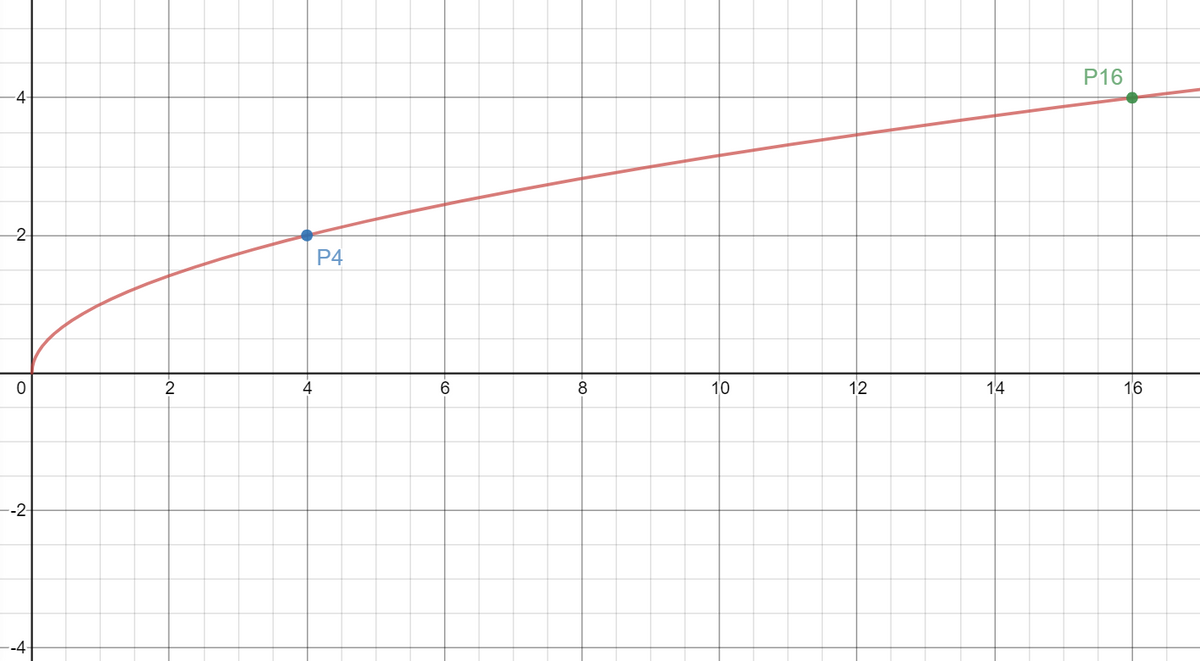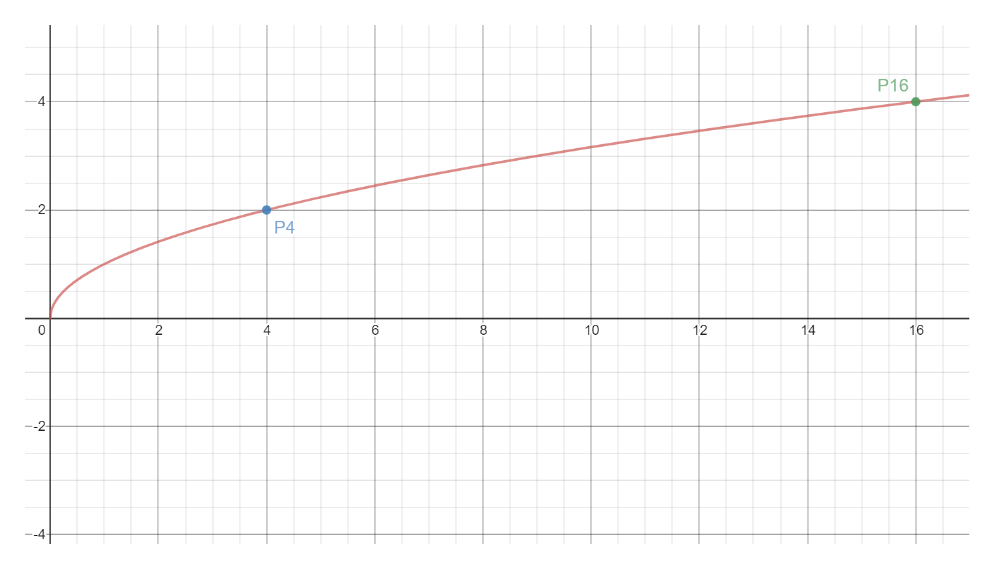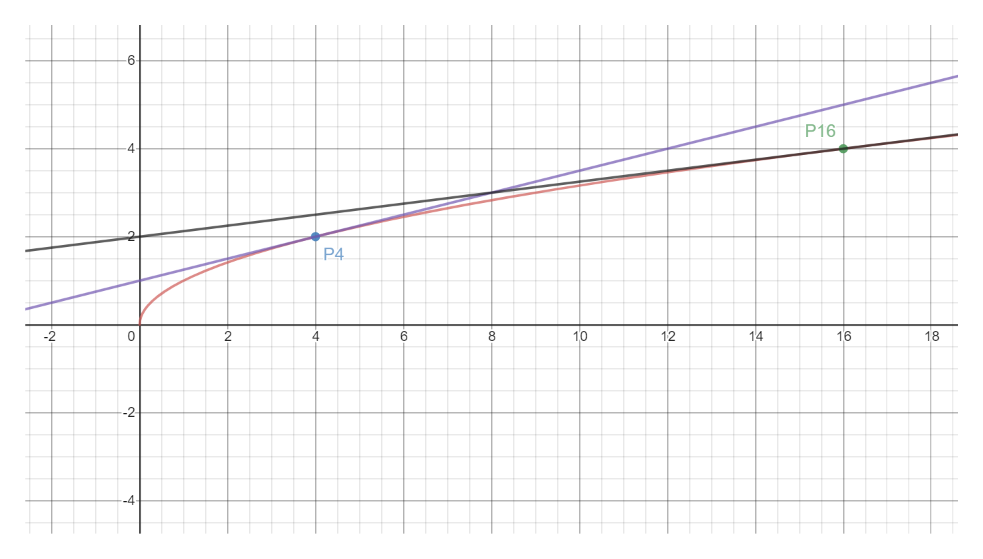Graph y = √x on a sheet of paper.  Label the points x = 4 and x = 16 on the x –axis.  Find the corresponding point on the curvy y = √x at x = 4 (Call this Point P4) and x = 16 (Call this Point P16).  Draw the tangent lines at P4 and P16. Compare the tangent lines you constructed. a.The slope of the tangent at x = 4 is less than the slope of the tangent at x = 16 b.The slope of the tangent at x = 4 is greater than the slope of the tangent at x = 16 c.The slope of the tangent at x = 4 is equal to the slope of the tangent at x = 16 d.The slope of the tangent at x = 4 exist but the slope of the tangent at x = 16 does not exist. e.None of these

Question

Graph y = √x on a sheet of paper.  Label the points x = 4 and x = 16 on the x –axis.  Find the corresponding point on the curvy y = √x at x = 4 (Call this Point P4) and x = 16 (Call this Point P16).  Draw the tangent lines at P4 and P16. Compare the tangent lines you constructed.

 a. The slope of the tangent at x = 4 is less than the slope of the tangent at x = 16 b. The slope of the tangent at x = 4 is greater than the slope of the tangent at x = 16 c. The slope of the tangent at x = 4 is equal to the slope of the tangent at x = 16 d. The slope of the tangent at x = 4 exist but the slope of the tangent at x = 16 does not exist. e. None of these
Step 1

When x=4 then y=sqrt(4)=2

So point is (4,2)

Step 2

When x=16 then y=sqrt(16)=4

So, point is (16,4)help_outlineImage TranscriptioncloseP16 P4 0 2 4 6 8 10 12 14 16 --2 fullscreen
Step 3

Then we draw tangents at P4 and P16.

The violet line is t...help_outlineImage Transcriptionclose6 P16 P4 12 10 14 16 0 2 4 6 18 fullscreen

Want to see the full answer?

See Solution

Want to see this answer and more?

Our solutions are written by experts, many with advanced degrees, and available 24/7

See Solution
Tagged in

Other